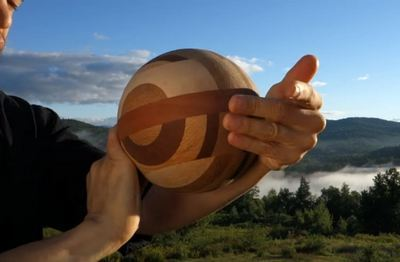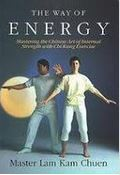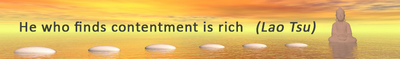Like   Tweet   Pin   +1   in/* styles */ Performance + Wellbeing; Stories; Tips and Resources   ||   Issue #33 January 2021
 table div table+table+table div table{width:100%;padding:0}table div table+table+table div table img{width:96.23%;padding:0;float:none}table div table+table+table div table td{width:100%;padding:0 1.88% 18px}/* styles */I hope you had a good start to the year. I've had a complete 'vegie' break' over Christmas and New Year and starting to get back into the swing of things.

This year I've decided to rebrand this e-zine and call it Sports Chi as I'm focusing more on the sports side of my business. I haven't got the time to run two separate e-zines.

There won't be much change in the content you have already received as I will continue to post Tai Chi related wellbeing and performance tips and resources. The only addition will be a couple of short sports related posts and/or resources.

Regards
Chris Bennett
Chris Chi

 table div table+table+table+table+table div table{width:100%;padding:0}table div table+table+table+table+table div table img{width:96.23%;padding:0;float:none}table div table+table+table+table+table div table td{width:100%;padding:0 1.88% 18px}/* styles */I was training my low stance the other day and my legs were getting wobbly and to achieve my target I had the choice of either using will power or letting go and just relaxing my mind.

Well, I just let go of my racing mind, relaxed and achieved my target.

In my earlier days I used will power to get through difficult Tai Chi exercises but that was counter productive and more tiring than training your mind to let go.

So if you find yourself using will power to get thorough a difficult exercise learn how to 'let yourself go' and you'll be surprised on what you can achieve.

Image courtesy of Billy Pasco via Unsplash

 table div table+table+table+table+table+table+table+table div table{width:100%;padding:0}table div table+table+table+table+table+table+table+table div table img{width:96.23%;padding:0;float:none}table div table+table+table+table+table+table+table+table div table td{width:100%;padding:0 1.88% 18px}/* styles */## Tai Chi Tip

 /* styles */ To straightened and relax your neck keep your chin slightly in
 table div table+table+table+table+table+table+table+table+table+table+table div table{width:100%;padding:0}table div table+table+table+table+table+table+table+table+table+table+table div table img{width:96.23%;padding:0;float:none}table div table+table+table+table+table+table+table+table+table+table+table div table td{width:100%;padding:0 1.88% 18px}/* styles */## Tai Chi Ball ExerciseA short clip of Adison Martin from YMMA **demonstrating a Tai Chi ball exercise. I've practiced Tai Chi ball exercisess and they are good workout.

I've used balls the size of a soccer or basketball to work out but you can use smaller or larger ones if you prefer. To get a good Tai Chi benefit make sure your stance is correct (high or mid level) and turn your waist as you move your hands.

 table div table+table+table+table+table+table+table+table+table+table+table+table+table+table div table{width:100%;padding:0}table div table+table+table+table+table+table+table+table+table+table+table+table+table+table div table img{width:96.23%;padding:0;float:none}table div table+table+table+table+table+table+table+table+table+table+table+table+table+table div table td{width:100%;padding:0 1.88% 18px}/* styles */## The Way of EnergyThe Way of Energy **by Master Lam Kam Chuen is a well designed, Illustrated and easy to to read book. He covers ithe benefits of Chi (energy) and has several exercises suitable for beginners.

It's divided in four parts and chapters include; Learning to Stand - Breathing and relaxing - Internal Movement - Preparing for Energy - Growing like a Tree - Roots and Branches - Going Beyond - Energy in Daily Life - Taking Care of Yourself.

I use aspects of this book as a quick reference guide when I'm researching a theme on Chi (energy).

It's priced at \$32.93 on Book Depository and is worth the price.

 table div table+table+table+table+table+table+table+table+table+table+table+table+table+table+table+table+table div table{width:100%;padding:0}table div table+table+table+table+table+table+table+table+table+table+table+table+table+table+table+table+table div table img{width:96.23%;padding:0;float:none}table div table+table+table+table+table+table+table+table+table+table+table+table+table+table+table+table+table div table td{width:100%;padding:0 1.88% 18px}/* styles */table div table+table+table+table+table+table+table+table+table+table+table+table+table+table+table+table+table+table+table div table{width:100%;padding:0}table div table+table+table+table+table+table+table+table+table+table+table+table+table+table+table+table+table+table+table div table img{width:96.23%;padding:0;float:none}table div table+table+table+table+table+table+table+table+table+table+table+table+table+table+table+table+table+table+table div table td{width:100%;padding:0 1.88% 18px}/* styles *//* styles */
 table div table+table+table+table+table+table+table+table+table+table+table+table+table+table+table+table+table+table+table+table+table div table{width:100%;padding:0}table div table+table+table+table+table+table+table+table+table+table+table+table+table+table+table+table+table+table+table+table+table div table img{width:96.23%;padding:0;float:none}table div table+table+table+table+table+table+table+table+table+table+table+table+table+table+table+table+table+table+table+table+table div table td{width:100%;padding:0 1.88% 18px}/* styles *//* styles */